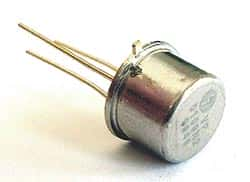# VP0109N2 P-Channel MosFETManufacturer: Supertex
Part Number: VP0109N2
Package: TO-39

Price: \$2.00
4242 available for immediate delivery
Code: T192PD
Volume Pricing
Quantity Price Each
1-9\$2.00
10-99\$1.95
100+\$1.85
Quantity:

MOS FET
Enhancement Mode P-Channel Mosfet
Obsolete - Replaced by VP2206N2

Specs:
V(BR)DSS (V)=90
V(BR)GSS (V)=20
I(D) Abs. Drain Current (A)=450m
I(DM) Max (A)(@25�C)=1.0
@Pulse Width (s) (Condition)=300u
Absolute Max. Power Diss. (W)=3.5
Thermal Resistance Junc-Amb.=125
V(GS)th Max. (V)=3.5
V(GS)th Min. (V)=1.5
@(VDS) (V) (Test Condition)=20
@I(D) (A) (Test Condition)=1.0m
I(DSS) Max. (A)=10u
@V(DS) (V) (Test Condition)=90
@Temp (�C) (Test Condition)=25
I(GSS) Max. (A)=100n
@V(GS) (V) (Test Condition)=20
r(DS)on Max. (Ohms)=15
@V(GS) (V) (Test Condition)=5.0
@I(D) (A) (Test Condition)=100m
g(fs) Min. (S) Trans. conduct.=150m
g(fs) Max (S) Trans. conduct=190m
@V(DS) (V) (Test Condition)=25
@I(D) (A) (Test Condition)=500m
C(iss) Max. (F)=60p
@V(DS) (V) (Test Condition)=25
@Freq. (Hz) (Test Condition)=1.0M
td(on) Max (s) On time delay=6.0n
t(r) Max. (s) Rise time=10n
t(d)off Max. (s) Off time=7.0n
t(f) Max. (s) Fall time.=10n
Status=Discontinued
Package=TO-39

Manufactured by: Supertex
Part Number: VP0109N2

Full Datasheet: VP0109N2